# Exploratory data analysis: N. Y. CityCabs data: 2009-2015¶

In :
import vaex
from vaex.ui.colormaps import cm_plusmin

import numpy as np
import pylab as plt
import seaborn as sns

import warnings
warnings.filterwarnings("ignore")


### Adjusting matplotlib parameters¶

In :
SMALL_SIZE = 12
MEDIUM_SIZE = 14
BIGGER_SIZE = 16

plt.rc('font', size=SMALL_SIZE)          # controls default text sizes
plt.rc('axes', titlesize=SMALL_SIZE)     # fontsize of the axes title
plt.rc('axes', labelsize=MEDIUM_SIZE)    # fontsize of the x and y labels
plt.rc('xtick', labelsize=SMALL_SIZE)    # fontsize of the tick labels
plt.rc('ytick', labelsize=SMALL_SIZE)    # fontsize of the tick labels
plt.rc('legend', fontsize=SMALL_SIZE)    # legend fontsize
plt.rc('figure', titlesize=BIGGER_SIZE)  # fontsize of the figure title


### Obtaining the data¶

The original data is courtesy of the New York City Taxi and Limousine Commision, and can be downloaded from this website.

The data was then converted to the memory-mappable HDF5 file format. For an example on how to do this, you may want to look at this notebook.

• Can "read" the memmory mapped file that we have on disk in no time.
• Vaex can also read data stored on S3. The data is streamed on need-to-have basis and is locally cached.
In :
# Check the file size on disk
!ls -l -h ./data/yellow_taxi_2009_2015_f32.hdf5

-rw-r--r--  1 jovan  staff   107G Jul  3 12:36 ./data/yellow_taxi_2009_2015_f32.hdf5

In :
# Read in the data from disk
df = vaex.open('./data/yellow_taxi_2009_2015_f32.hdf5')

In :
# # Read in the data from S3
# df = vaex.open('s3://vaex/taxi/yellow_taxi_2009_2015_f32.hdf5?anon=true')

In :
# A view into the data
df

Out:
# vendor_id pickup_datetime dropoff_datetime passenger_count payment_type trip_distance pickup_longitude pickup_latitude rate_code store_and_fwd_flag dropoff_longitude dropoff_latitude fare_amount surcharge mta_tax tip_amount tolls_amount total_amount
0 VTS 2009-01-04 02:52:00.0000000002009-01-04 03:02:00.0000000001 CASH 2.630000114440918 -73.9919586181640640.72156524658203 nan nan -73.99380493164062 40.6959228515625 8.899999618530273 0.5 nan 0.0 0.0 9.399999618530273
1 VTS 2009-01-04 03:31:00.0000000002009-01-04 03:38:00.0000000003 Credit 4.550000190734863 -73.9821014404296940.736289978027344nan nan -73.95584869384766 40.76802825927734412.1000003814697270.5 nan 2.0 0.0 14.600000381469727
2 VTS 2009-01-03 15:43:00.0000000002009-01-03 15:57:00.0000000005 Credit 10.350000381469727-74.0025863647461 40.73974609375 nan nan -73.86997985839844 40.77022552490234423.7000007629394530.0 nan 4.739999771118164 0.0 28.440000534057617
3 DDS 2009-01-01 20:52:58.0000000002009-01-01 21:14:00.0000000001 CREDIT 5.0 -73.9742660522461 40.79095458984375 nan nan -73.9965591430664 40.73184967041015614.8999996185302730.5 nan 3.049999952316284 0.0 18.450000762939453
4 DDS 2009-01-24 16:18:23.0000000002009-01-24 16:24:56.0000000001 CASH 0.4000000059604645-74.0015792846679740.719383239746094nan nan -74.00837707519531 40.7203483581543 3.700000047683716 0.0 nan 0.0 0.0 3.700000047683716
... ... ... ... ... ... ... ... ... ... ... ... ... ... ... ... ... ... ...
1,173,057,922VTS 2015-12-31 23:59:56.0000000002016-01-01 00:08:18.0000000005 1 1.2000000476837158-73.9938125610351640.72087097167969 1.0 0.0 -73.98621368408203 40.7224693298339847.5 0.5 0.5 1.75999999046325680.0 10.5600004196167
1,173,057,923CMT 2015-12-31 23:59:58.0000000002016-01-01 00:05:19.0000000002 2 2.0 -73.9652709960937540.76028060913086 1.0 0.0 -73.93951416015625 40.75238800048828 7.5 0.5 0.5 0.0 0.0 8.800000190734863
1,173,057,924CMT 2015-12-31 23:59:59.0000000002016-01-01 00:12:55.0000000002 2 3.799999952316284 -73.9872970581054740.7390785217285161.0 0.0 -73.9886703491211 40.69329833984375 13.5 0.5 0.5 0.0 0.0 14.800000190734863
1,173,057,925VTS 2015-12-31 23:59:59.0000000002016-01-01 00:10:26.0000000001 2 1.9600000381469727-73.99755859375 40.72569274902344 1.0 0.0 -74.01712036132812 40.705322265625 8.5 0.5 0.5 0.0 0.0 9.800000190734863
1,173,057,926VTS 2015-12-31 23:59:59.0000000002016-01-01 00:21:30.0000000001 1 1.059999942779541 -73.9843978881836 40.76725769042969 1.0 0.0 -73.99098205566406 40.76057052612305 13.5 0.5 0.5 2.96000003814697270.0 17.760000228881836

### Quick insights into this dataset¶

This is done with a single pass over the data

In :
# Get a high level overview of the DataFrame
df.describe()

Out:
vendor_id pickup_datetime dropoff_datetime passenger_count payment_type trip_distance pickup_longitude pickup_latitude rate_code store_and_fwd_flag dropoff_longitude dropoff_latitude fare_amount surcharge mta_tax tip_amount tolls_amount total_amount
dtype str datetime64[ns] datetime64[ns] int64 str float32 float32 float32 float32 float32 float32 float32 float32 float32 float32 float32 float32 float32
count 1173057927 1173057927 1173057927 1173057927 1173057927 1173057927 1173057927 1173057926 1002161871 638914438 1173043432 1173050240 1173057925 1173057925 1032017356 1173057925 1173057925 1173057925
NA 0 0 0 0 0 0 0 1 170896056 534143489 14495 7687 2 2 141040571 2 2 2
mean -- 1970-01-01T00:00:01.953533625 1970-01-01T00:00:14.506598422 1.6844313554517245 -- 5.390923660971188 -72.53224844702918 39.934531393518846 1.035820754150404 0.017168377090266976 -72.53741806425039 39.93694872311044 11.217308155800902 0.3036385232379654 0.4963069205116384 1.1294571893026348 0.18678067517758123 13.314765814201273
std -- 6.22239e+16 6.22266e+16 1.33032 -- 7756.52 12.7505 9.51675 0.441996 0.129899 12.6768 9.50487 633.505 0.395407 0.0683994 132.842 886.718 1098.43
min -- 2009-01-01T00:00:27.365015552 1899-12-31T23:59:43.370698752 0 -- -4.08401e+07 -3509.02 -3579.14 0 0 -3579.14 -3579.14 -2.14748e+07 -79 -3 -1.67772e+06 -2.14748e+07 -2.14748e+07
max -- 2016-01-01T00:00:49.632313344 2253-08-23T08:00:13.061652480 255 -- 1.98623e+08 3570.22 3577.14 252 2 3460.43 3577.14 825999 999.99 1311.22 3.95059e+06 5510.07 3.95061e+06

### Getting read of outliers and errouneous data¶

In this section we will use the output of describe to get rid of outliers, and other erroneous data. Let's start with the City of New York itself.

Let's visualise the pickup locations.

In :
# Interactively visualise the pickup locations of all taxi trips in our dataset.
df.plot_widget(df.pickup_longitude,
df.pickup_latitude,
shape=512,
limits='minmax',
f='log1p',
colormap='plasma')

Plot2dDefault(w=None, what='count(*)', x='pickup_longitude', y='pickup_latitude', z=None)

With Vaex we can interactively explore such heamaps as the one above, even when the data contains over 1 billion samples. This way we can choose the spatial extent over which the taxi company operates in New York City. In fact, it is mostly Manhattan.

In :
# Define the boundaries by interactively choosing the area of interest!
long_min = -74.05
long_max = -73.75
lat_min = 40.58
lat_max = 40.90

# Make a selection based on the boundaries
df_filtered = df[(df.pickup_longitude > long_min)  & (df.pickup_longitude < long_max) & \
(df.pickup_latitude > lat_min)    & (df.pickup_latitude < lat_max) & \
(df.dropoff_longitude > long_min) & (df.dropoff_longitude < long_max) & \
(df.dropoff_latitude > lat_min)   & (df.dropoff_latitude < lat_max)]


From the output of the describe method we see that the maximum number of passengers is 255! Let's make a bar plot showing the common number of passengers in a taxi trip.

In :
# Get number of unique trips with certain number of passengers
num_passengers = df_filtered.passenger_count.value_counts(progress=True)

# Plot the result
plt.figure(figsize=(16, 4))
sns.barplot(x=num_passengers.index, y=np.log10(num_passengers.values))
plt.xlabel('Number of passengers')
plt.ylabel('Number of trips [dex]')
plt.xticks(rotation='45')
plt.show()

[########################################]:  100.00% elapsed time  :       23s =  0.4m =  0.0h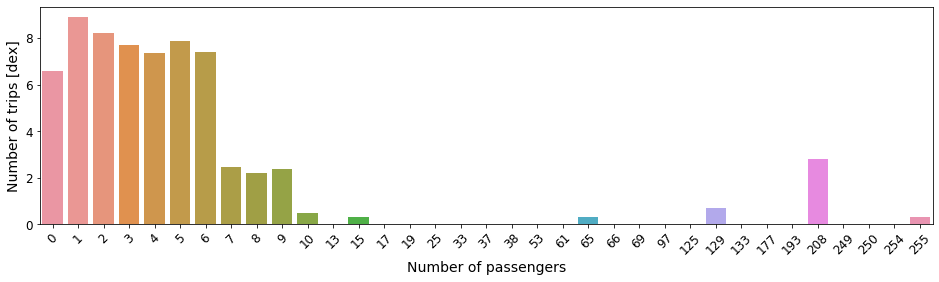First impressions: Typical number of passengers in a ride between 1-6. Very large number of taxi trips with 0 passengeres. Are these deliveries maybe, or were passengers not recorded. There are few hundreds of taxi trips with 7-9 passengers, and beyond that the numbers look erroneous.

In this analysis we will focus only on the trips with typical number of passengers, that is between 1 and 6. So let's add that do the filter.

In :
# Filterd based on the number of passengers
df_filtered = df_filtered[(df_filtered.passenger_count>0) & (df_filtered.passenger_count<7)]


Next up, we turn to the distance column. Here we see that the minimum value is negative, i.e. for sure something has gone wrong, and the maximum values is.. well very large! In fact, to put this in perspective:

In :
# What is the largest distance?
max_trip_distance = df_filtered.trip_distance.max().astype('int')

print(f'The largest distance in the data is {max_trip_distance} miles!')

print(f'This is {max_trip_distance/238_900:.1f} times larger than the distance between the Earth and the Moon!')

The largest distance in the data is 198623008 miles!
This is 831.4 times larger than the distance between the Earth and the Moon!


Let's plot the distribution of distances, but in a more sensible range, relative to the scale of the part of New York City we selected above.

In :
# Plot the distribution of distances.
plt.figure(figsize=(8, 4))
df_filtered.plot1d('trip_distance', limits=[0, 250], f='log10', shape=128, lw=3, progress=True)
plt.xlabel('Trip distance [miles]')
plt.ylabel('Number of trips [dex]')
plt.show()

[########################################]:  100.00% elapsed time  :        0s =  0.0m =  0.0h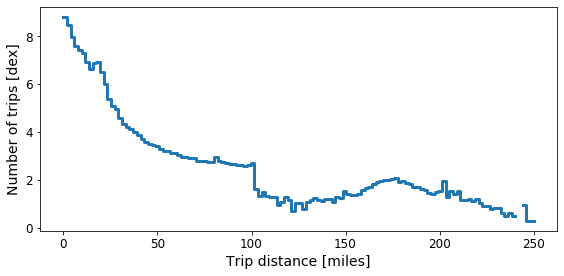So we observe that at ~100 miles, the number of taxi trips drops considerably, and becomes more sporadic. Thus we decide to only consider taxi trip that in total are up to 100 miles.

In :
# Select taxi trips have travelled maximum 100 miles (but also with non-zero distance).
df_filtered = df_filtered[(df_filtered.trip_distance > 0) & (df_filtered.trip_distance < 100)]


In the next step of our data cleaning process, let's look at the distributions of trip times and speeds, and make sure they are sensible. These quantities are not readily available in the dataset, but are trivial to compute. We will do this in a rather standard way, but here is the kick: these additional columns do not cost any memory what so ever. This is what we call virtual columns.

In :
# Time in transit (minutes)
df_filtered['trip_duration_min'] = (df_filtered.dropoff_datetime - df_filtered.pickup_datetime) / \
np.timedelta64(1, 'm')

# Speed (miles per hour)
df_filtered['trip_speed_mph'] = df_filtered.trip_distance / \
((df_filtered.dropoff_datetime - df_filtered.pickup_datetime) / \
np.timedelta64(1, 'h'))

In :
# Plot the distribution of trip durtaions
plt.figure(figsize=(8, 4))
df_filtered.plot1d('trip_duration_min', limits=[0, 600], f='log10', shape=64, lw=3, progress=True)
plt.xlabel('Trip duration [minutes]')
plt.ylabel('Number of trips [dex]')
plt.show()

[########################################]:  100.00% elapsed time  :       24s =  0.4m =  0.0h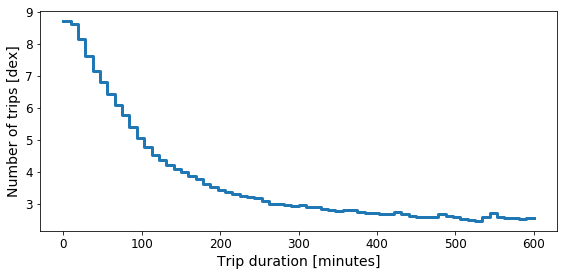We see that the majority of taxi trips, 95% to be exact last less than 30 minutes. From the above plot, we see the distribution falls of, and almost becomes flat after 200 minutes. Can you imagine, spending over 3 hours in a taxi in New York City! Perhaps it happens..

So let's be..open minded for now, and consider all trips that last less than 3 hours in total.

In :
# Filter taxi trips that have unreasonably long dirations
df_filtered = df_filtered[(df_filtered.trip_duration_min > 0) & (df_filtered.trip_duration_min < 180)]


Now let's look at the mean speed of a trip. Let us first look a the extremes:

In :
# Minimum and maximum average speed of a taxi trip
print('Minimal mean speed: %.3f miles/hour.' % (df_filtered.trip_speed_mph.min()))
print('maximal mean speed: %.3f miles/hour.' % (df_filtered.trip_speed_mph.max()))

Minimal mean speed: 0.003 miles/hour.
maximal mean speed: 234359.995 miles/hour.


From the extremes of this column we notice that we have some serious outliers. On the lower end of the spectrum, the slowest speeds are considerably slower than walking sleeds. On the high end of the spectrum, those cars are flying so fast, they can be used as spaceships!

Let us plot the distribution of mean speeds, for a more sensible, or at least physically viable range.

In :
# Plot the distribution of trip durtaions
plt.figure(figsize=(8, 4))
df_filtered.plot1d('trip_speed_mph', limits=[0, 120], f='log10', shape=64, lw=3, progress=True)
plt.xlabel('Mean speed during a trip [miles/hour]')
plt.ylabel('Number of trips [dex]')
plt.show()

[########################################]:  100.00% elapsed time  :        7s =  0.1m =  0.0h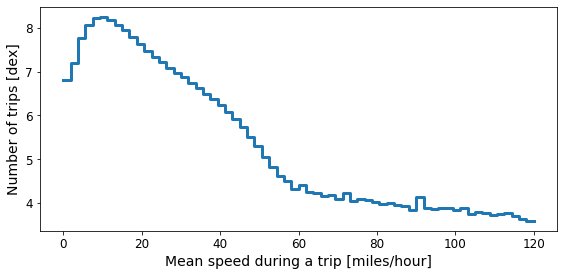Based on this plot we can make a sensible choce of a typical trip speed: somewhere in the range of 1-60 miles per hour.

In :
# Filter out errouneous average trip speeds.
df_filtered = df_filtered[(df_filtered.trip_speed_mph > 1) & (df_filtered.trip_speed_mph < 60)]


Finally, let's look at the cost of the taxi trips. From the output of the describe() function, we can see that there are some crazy outliers in the fare_amount, total_amount, and tip_amount. For starters, no value in these columns should be negative. Also their upper limits are ridiculously high. Let's look at their distributions, but in a more sensible range.

In :
plt.figure(figsize=(18, 5))

plt.subplot(131)
df_filtered.plot1d('fare_amount', shape=64, lw=3, limits=[0, 1000], f='log10', progress=True)
plt.xlabel('Fare amount [$]') plt.ylabel('Number of trips [dex]') plt.tick_params(labelsize=14) plt.subplot(132) df_filtered.plot1d('total_amount', shape=64, lw=3, limits=[0, 1000], f='log10', progress=True) plt.xlabel('Total amount [$]')
plt.ylabel('')
plt.tick_params(labelsize=14)

plt.subplot(133)
df_filtered.plot1d('tip_amount', shape=64, lw=3, limits=[0, 1000], f='log10', progress=True)
plt.xlabel('Tip amount [$]') plt.ylabel('') plt.tick_params(labelsize=14) plt.tight_layout() plt.show()  [########################################]: 100.00% elapsed time : 19s = 0.3m = 0.0h [########################################]: 100.00% elapsed time : 5s = 0.1m = 0.0h [########################################]: 100.00% elapsed time : 5s = 0.1m = 0.0h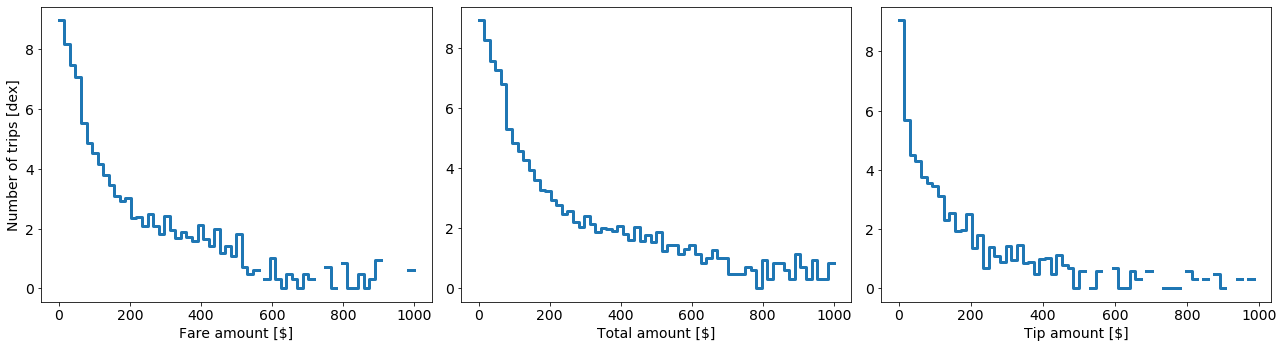We see that in all three cases, these distribution have some very long tail. Perhaps few of these large fares are legit, most are probably or hopefully errouneous data, or maybe some funny business is going on from time to time. In any case, we would like to focus on the regular "vanilla" rides, so we will select all trips that have total and fare amount less than \$200 (the elbow of the distributions). Same for the tips. Note that the tips are not included in the total amount column. We also require that the fare and total amount be larger than \\$0. This condition is not imposed on the tips, although none of these can be negative. In : df_filtered = df_filtered[((df_filtered.total_amount > 0) & (df_filtered.total_amount < 200) & (df_filtered.fare_amount > 0) & (df_filtered.fare_amount < 200) & (df_filtered.tip_amount >= 0) & (df_filtered.tip_amount < 200))]  Finally, after this initial cleaning of the data is done, let's see how many taxi trips we have left In : N_samples = len(df_filtered) print(f'Number of trips in the filtered dataset: {N_samples}')  Number of trips in the filtered dataset: 1129396332  We have over 1.1 billion taxi trips for our upcoming analysis. Let's get to it! ### General Exploratory Data Analysis¶ Let's assume we are a prospective taxi driver, or even a manager of a taxi company, and are interested in finding out where are, on average, the best hotspots to pick up passengers from, which will lead to large taxi fees. Naively, we can just plot a map of the pickup locations color-coded by the average fare amount for that big, i.e. part of the town. However, as a taxi driver, we have our own expences as well. We need to pay for fuel, or taking a passenger somewhere remote might mean that we will spend a lot of time and fuel just getting back to the city centre, and perhaps it will not be so easy to find a passenger for our trip back. Having that into consideration, we decide to instead color code the map of NYC by the mean of the trip fare divided by the trip distance. This is simple way we can introduce normalization, i.e. taking some of our costs into account. These two cases are plotted below In : # Define new columns that might prove useful: df_filtered['tip_percentage'] = df_filtered.tip_amount / df_filtered.total_amount * 100. df_filtered['fare_over_distance'] = df_filtered.fare_amount / df_filtered.trip_distance  In : plt.figure(figsize=(15, 5)) plt.subplot(121) df_filtered.plot('pickup_longitude', 'pickup_latitude', what='mean(fare_amount)', colormap='plasma', f='log1p', shape=512, colorbar=True, colorbar_label='mean fare amount [$]', vmin=1, vmax=4.5)

plt.xlabel('pickup longitude')
plt.ylabel('pickup latitude')

plt.subplot(122)
df_filtered.plot('pickup_longitude', 'pickup_latitude', what='mean(fare_over_distance)',
colormap='plasma', f='log1p', shape=512, colorbar=True,
colorbar_label='mean fare/distance [\$/mile]', vmin=0.75, vmax=2.5)

plt.xlabel('pickup longitude')
plt.ylabel('')
plt.gca().axes.get_yaxis().set_visible(False)

plt.tight_layout()
plt.show()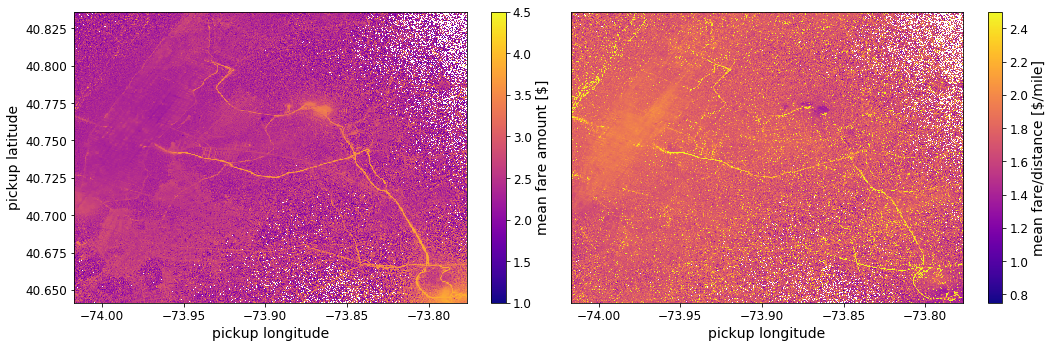We see that in the 1st case, if we just care about getting the maximum fare for the service provided, it is best to pick up passengers around the NYC airprots, and long the main streets, such as the Van Wyck Expressway, and Long Island Expressway avenues.

However, when we divide by the distance travelled, we get a slightly different picture. The Van Wyck Expressway, and Long Island Expressway avenues are still relevant, but much less prominant, the airports are not as popular. Some other hotspots appear on the other side of the Hudson river, which seem quite profitable locations to pick up passengers from.

##### When do trips happens¶

Next, we wanna figure out when do most of the taxi usage happens so we can schedule our working hours appropriately.

In :
# Extract some date/time features
df_filtered['pickup_hour'] =